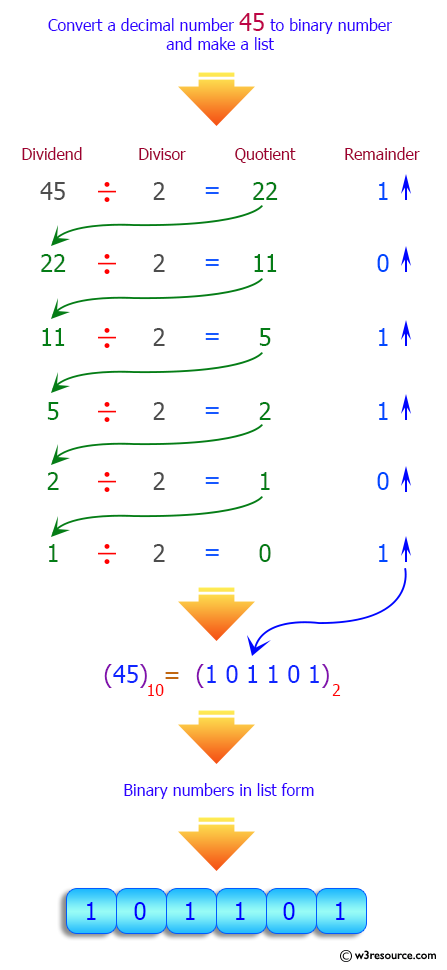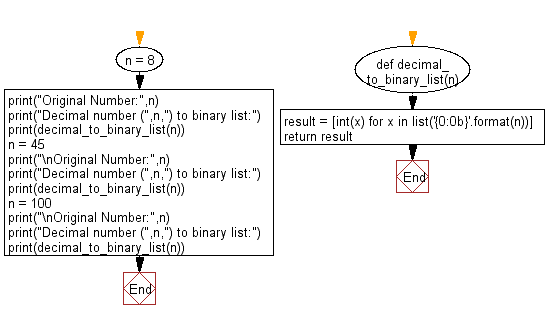﻿ Python: Convert a given decimal number to binary list - w3resource# Python: Convert a given decimal number to binary list

## Python List: Exercise - 185 with Solution

Write a Python program to convert a given decimal number to binary list.

Sample Solution:

Python Code:

``````def decimal_to_binary_list(n):
result = [int(x) for x in list('{0:0b}'.format(n))]
return result
n = 8
print("Original Number:",n)
print("Decimal number (",n,") to binary list:")
print(decimal_to_binary_list(n))
n = 45
print("\nOriginal Number:",n)
print("Decimal number (",n,") to binary list:")
print(decimal_to_binary_list(n))
n = 100
print("\nOriginal Number:",n)
print("Decimal number (",n,") to binary list:")
print(decimal_to_binary_list(n))
```
```

Sample Output:

```Original Number: 8
Decimal number ( 8 ) to binary list:
[1, 0, 0, 0]

Original Number: 45
Decimal number ( 45 ) to binary list:
[1, 0, 1, 1, 0, 1]

Original Number: 100
Decimal number ( 100 ) to binary list:
[1, 1, 0, 0, 1, 0, 0]
```

Pictorial Presentation:Flowchart:## Visualize Python code execution:

The following tool visualize what the computer is doing step-by-step as it executes the said program:

Python Code Editor:

Have another way to solve this solution? Contribute your code (and comments) through Disqus.

What is the difficulty level of this exercise?

Test your Python skills with w3resource's quiz

﻿

## Python: Tips of the Day

```print(2_000_000)
```2000000